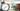# How to calculate angle between hour and minute hand, given a time

August 31, 2019This problem is a simple mathematical calculation. Lets start deriving some equations. Note: Here we are talking about the round clock, which displays 1-12 hours.

## Angle of Minute hand at a particular time

A complete round of a clock is equal to 360 degree angle. This is simple, minute hand complete 360 degree in 60 minutes. Or, it takes 60 minutes to complete 360 degree

``````60 M -> 360 degree
1 M -> 360/60 -> 6 degree

Means, minute hands moves 6 degree per minute.

For 'M' minutes, it will be 6 * M degree``````

## Angle of Hour hand at a particular time

Hour hand takes 12 hours to complete a round i.e. 360 degree. But, if you notice. When hour hand moves, there will be a movement in minute hand too, due to that hour hand will move to some extent.

``````For hour hand:
12 Hours -> 360 degree
1 Hour -> 360/12 -> 30 degree

For 'H' Hours -> 30 * H degree

And, there will be some movement in minute hand too. Continuing above equation:
12 Hours -> 360 degree
12 * 60 Minutes -> 360 degree
1 Minute -> 360 / (12 * 60) -> 1/2 degree

In 'M' minuets -> M/2 degree

Combining them, Angle of hour : 30 H + M/2``````

## Difference of Angle between Hour and Minute hand

As from above equations:

``````Angle of Hour hand - Angle of Minute hand
=> (30 H + M/2) - (6 M)
=> 30 H - 5.5 M``````

## Example

Angle at time: 6:15

``````30 H - 5.5 M
=> 30 * 6 - 5.5 * 15
=> 180 - 82.5
=> 97.5``````